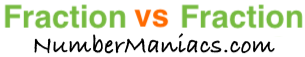2/3 vs 1/2People compare 2/3 vs 1/2 drywall, 2/3 vs 1/2 pipe, 2/3 vs 1/2 cup, 2/3 vs 1/2 ton, 2/3 vs 1/2 drill bit, 2/3 vs 1/2 poker, 2/3 vs 1/2 sump pump, 2/3 vs 1/2 garden hose, and many other items.

Is 2/3 greater than 1/2? Is 2/3 less than 1/2? What is the difference between 2/3 and 1/2? These and other questions are answered below so you can get a better perspective of 2/3 vs 1/2.

2/3 vs 1/2 Fractional Comparisons
2/3 is more than 1/2
The difference between 2/3 and 1/2 is 1/6
2/3 is 1/6 more than 1/2

2/3 vs 1/2 Decimal Comparisons
2/3 vs 1/2 as a decimal is 0.667 vs 0.5
The difference between 2/3 and 1/2 is 0.167
2/3 is 0.167 more than 1/2

2/3 vs 1/2 Percent Comparisons
2/3 vs 1/2 as a percent is 66.7% vs 50%
2/3 is 33.333 percent more than 1/2

Fraction vs Fraction
Submit another set of fractions that we can compare for you!

/
vs
/

The math behind the numbers
Calculations on this page are rounded to the nearest thousandth if necessary. Below is the math we used to analyze 2/3 vs 1/2:

To compare the size of 2/3 vs 1/2, we simply subtracted 1/2 from 2/3:

2/3 - 1/2 = |1/6|

To find 2/3 vs 1/2 as a decimal, we divided the numerator by the denominator for each fraction.

2 ÷ 3 = 0.667
1 ÷ 2 = 0.5

To find 2/3 vs 1/2 as a percent, we divided the numerator by the denominator and then multiplied the quotient by 100 for each fraction.

(2 ÷ 3) x 100 = 66.7%
(1 ÷ 2) x 100 = 50%

We found that 2/3 is 33.333 percent more than 1/2 by dividing the difference between the two fractions by the second fraction and then multiplied it by 100:

((1/6)/(1/2))×100 = 33.333%

That's it folks. We hope this page gave you a better understanding of 2/3 vs 1/2 with our explanations and conclusions.

2/3 vs 1/3
Here are the next two fractions we have compared and analyzed.